# Resampler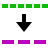The Resampler I/O resamples the source's data to decrease or increase the sample frequency (and record length with the same ratio) of a signal. This can be useful when several signals are sampled with a high sample frequency and record length but not all of them require this high speed and record length.

Hint: When using an RPM I/O to determine the speed of an engine, the sample frequency must be around 10 kHz or higher to get the accurate rpms. Once the speed has been determined, it can be resampled to a lower sample frequency, for example 10 Hz, because the speed will vary relatively slow. This decreases the amount of data (or file size) by a factor 1000.

## Properties and actions

To control the behavior of the Resampler I/O, several properties and actions are available. These can be accessed through a popup menu which is shown when the I/O is right clicked in the Object Tree. The properties can also be accessed through its settings window which is shown when the I/O is double clicked in the Object Tree. To open the Object tree, click the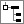Show object tree button.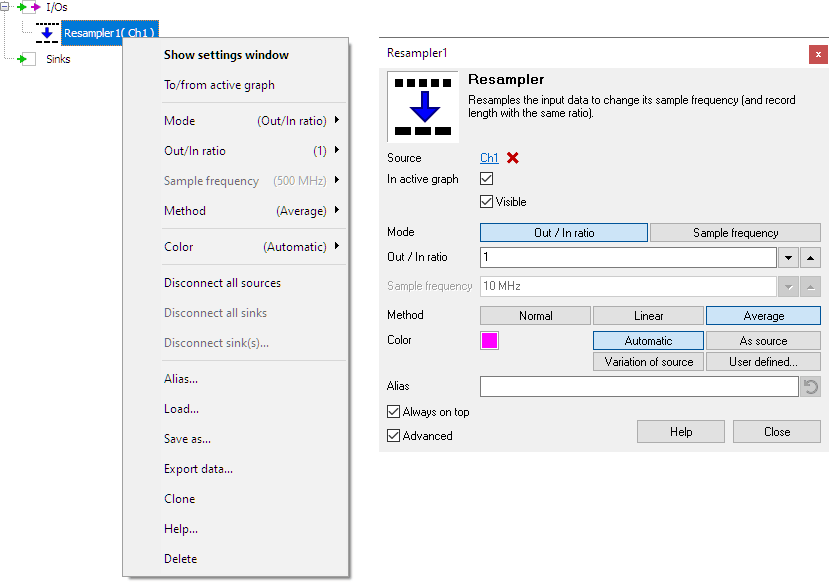By default, the settings window only shows the most used settings. When Advanced is ticked, the extended window with all settings is shown. See also the program settings.

### Mode

The Mode property determines how the Resampler I/O is setup: for a required out/in ratio or for a required output sample frequency.

#### Out / In ratio

The Out / In ratio property defines the ratio between the output sample frequency and the input sample frequency. Several standard values are available, as well as a user defined setting. When the sample frequency of the input signal changes, the sample frequency of the output signal will change accordingly.

#### Sample frequency

The Sample frequency property sets the output sample frequency of the Resampler I/O to the specified value. Several standard values are available, as well as a user defined setting. The Out/In ratio will be calculated automatically, based on the sample frequency of the input signal. When the sample frequency of the input signal changes, the sample frequency of the output signal will remain the same, a new ratio will be calculated.

### Method

The Method property determines how the data is resampled:

#### Normal

• When the ratio is smaller than 1, only one sample of the input data is used for each output sample.
• When the ratio is greater than 1, several samples in the output data are filled with each input sample.
 IN ratio OUT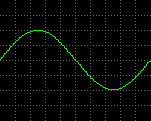→ 1/10 →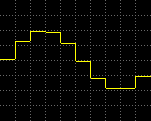100 Samples 10 Samples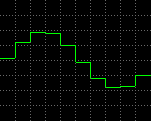→ x10 →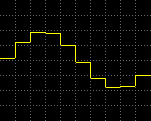10 Samples 100 Samples

#### Linear

• When the ratio is smaller than 1, only one sample of the input data is used for each output sample.
• When the ratio is greater than 1, the output data is a linear interpolation of the input data.
 IN ratio OUT→ 1/10 →100 Samples 10 Samples→ x10 →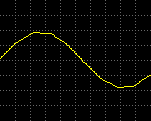10 Samples 100 Samples

#### Average

• When the ratio is smaller than 1, the mean value of several samples of the input data is used for each output sample.
• When the ratio is greater than 1, the output data is a linear interpolation of the input data.
 IN ratio OUT→ 1/10 →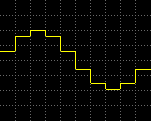100 Samples 10 Samples→ x10 →10 Samples 100 Samples

### Related information

#### RPM

The RPM I/O converts a crankshaft signal into revolutions per minute.